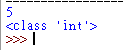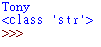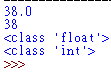#DAY 4
0

## Python的數值資料型態!

python的數值資料型態分為兩種

int 也是大家最熟的整數
float 就是浮點數(有小數點的那種)
bool 就是布林值true or false

``````i = 5
print(i)
print(type(i))
``````str 是字串需要用''或著""包起來的文字，是很多字元組成
chr 是字元 就是組成字串需要的元素

``````name = "Tony"
print(name)
print(type(name))
````````````floatex1 = 38.0
intex1 = int(floatex1)
print(floatex1)
print(intex1)
print(type(floatex1))
print(type(intex1))
``````